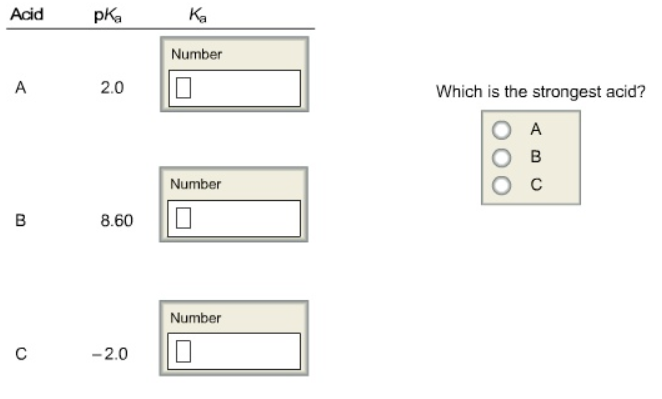# Problem: Determine the dissociation constants for the following acids. Express the answers in proper scientific notation where appropriate.

###### FREE Expert Solution

We are going to do the following steps

Step 1: Calculate Ka for each acid

Step 2: Determine the strongest acid

Step 1: Calculate Ka for each acid

Recall that we

98% (30 ratings)###### Problem Details

Determine the dissociation constants for the following acids. Express the answers in proper scientific notation where appropriate.